﻿ 基于概率分类模型的体数据分类可视化 Volume Data Classification Visualization Based on Probabilistic Classification Model

Computer Science and Application
Vol. 09  No. 11 ( 2019 ), Article ID: 32849 , 7 pages
10.12677/CSA.2019.911223

Volume Data Classification Visualization Based on Probabilistic Classification Model

Junda Zhang*, Xiao’an Tang

National University of Defense Technology, Changsha Hunan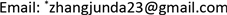Received: Oct. 16th, 2019; accepted: Oct. 31st, 2019; published: Nov. 7th, 2019ABSTRACT

Classification visualization is an important method for volume data study, which can intuitively reveal the structural characteristics. This paper proposes a classification visualization method based on probabilistic classification model. First, the voxel probabilistic classification model is established, which realizes the classification of voxel with the transfer function defined in gray histogram. Then voxel probabilistic classification model and kernel regression are combined to achieve more accurate reconstruction result, and the validity of reconstruction on reconstructed points is measured. Finally, from gray value to color and opacity, the visualization mapping based on reconstruction validity is implemented to obtain the final visualization result. The experiment shows that the proposed method can efficiently separate the feature structures of volume data and achieve a better classification visualization result of homogeneous continuity and heterogeneous separation.

Keywords:Volume Rendering, Ray Casting, Transfer Function, Classification Visualization, Kernel Regression1. 引言

2. 体素的概率分类模型

$p\left({\mathfrak{f}}_{k}|y\right),\text{\hspace{0.17em}}k=1,2,\cdots ,K$ (1)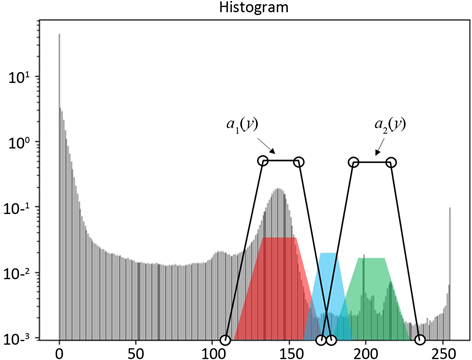Figure 1. Voxel probability classification based on gray histogram

$q\left({\mathfrak{f}}_{k}|y\right)=\frac{{\alpha }_{k}\left({y}_{i}\right)}{{\alpha }_{k\text{max}}},\text{\hspace{0.17em}}\text{\hspace{0.17em}}k=1,2,\cdots ,K$ (2)

$\begin{array}{l}p\left({\mathfrak{f}}_{k}|y\right)=\frac{q\left({\mathfrak{f}}_{k}|y\right)}{\text{max}\underset{k}{\sum }q\left({\mathfrak{f}}_{k}|y\right)}\\ p\left({\mathfrak{f}}_{0}|y\right)=1-\underset{k}{\sum }p\left({\mathfrak{f}}_{k}|y\right)\end{array}$ (3)

3. 面向分类的核回归重构

$\stackrel{^}{y}=\underset{{y}_{i}\in \Omega }{\sum }{\omega }_{i}{y}_{i}$ (4)

${\omega }_{i}=1-\frac{\text{Distance}\left(x,{x}_{i}\right)}{\sigma }$ (5)

${\stackrel{^}{y}}_{\mathfrak{f}}=\frac{\underset{{y}_{i}\in \Omega }{\sum }{\omega }_{i}{\omega }_{\mathfrak{f}}{y}_{i}}{\underset{{y}_{i}\in \Omega }{\sum }{\omega }_{i}{\omega }_{\mathfrak{f}}}$ (6)

4. 可视化映射

$\Phi \left(x,\mathfrak{f},\Omega \right)=\underset{{y}_{i}\in \Omega }{\sum }{\omega }_{i}{\omega }_{\mathfrak{f}}$ (7)

$\Phi \left(x,\mathfrak{f},\Omega \right)$ 可以描述为在特征 $\mathfrak{f}$ 的前提下，支撑域 $\Omega$ 范围内，对位置 $x$ 重构时的重构有效性。

$\begin{array}{l}{C}_{s}={C}_{s}+\left(1-{\alpha }_{s}\right){C}_{src}\\ {\alpha }_{s}={\alpha }_{s}+\left(1-{\alpha }_{s}\right){\alpha }_{src}\Phi \left(x,\mathfrak{f},\Omega \right)\end{array}$ (8)

5. 仿真结果及分析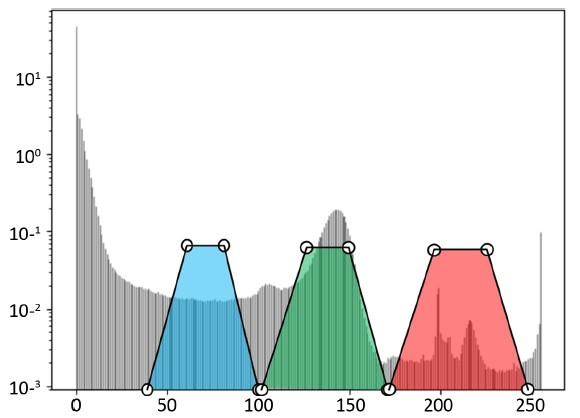Figure 2. The transfer function of Engine dataset

Engine数据的传递函数如图2所示，基于传递函数的分类方法采用基本的最小二乘重构，传递函数对应的最大颜色值和不透明度都为1。为了对比在相同的颜色映射下的体数据分类结果，本文算法也采用图2所示的传递函数，其中不透明度映射同时定义了三个特征类。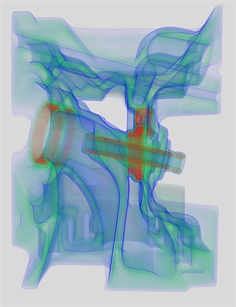Figure 3. The classification visualization result of Engine dataset

Engine数据的可视化结果如图3所示。基于传递函数的分类可视化方法(左图)由于无法实现体素的分类，仅仅从可视化映射阶段对体数据定义的特征类进行颜色和不透明度的映射。在重构过程中产生的大量中间灰度值，使分类可视化产生类似平滑滤波的结果，不同特征之间的空间关系被模糊，特征类不能被清晰地可视化表达。本文算法(右图)由于引入了体素的概率分类模型，实现了同质区域内连续，不同物质间分离的重构结果。同时，考虑到体数据空间分布的不均匀性，构造了能够结合体素概率分类模型的重构有效性 $\Phi \left(x,\mathfrak{f},\Omega \right)$。在进行可视化映射时，结合重构有效性进行光学属性的叠加，使最终的可视化结果能够清晰地可视化表达不同特征类。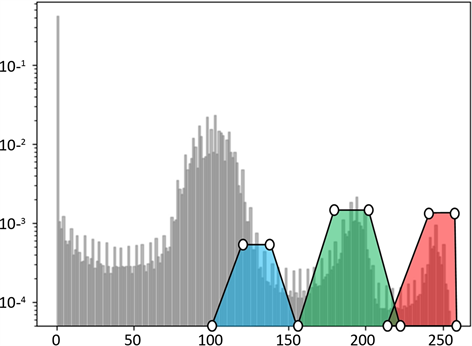Figure 4. The transfer function of Tooth dataset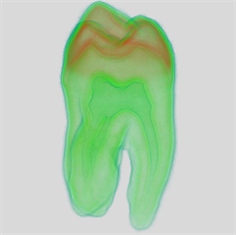Figure 5. The classification visualization result of Tooth dataset

Tooth数据的可视化结果如图5所示。与Engine数据的可视化结果类似，基于传递函数的分类可视化结果(左图)出现了特征类结构不清晰的问题，并且可见在牙齿结构表面覆盖的一层蓝色非有效数据出现许多“毛刺”，这是由传统重构方法产生的中间灰度值进入了用户定义的传递函数范围而引起的。本文算法(右图)的绘制结果中，蓝色的物质均匀地覆盖在牙齿结构表面，并且牙齿内部不同的特征类也非常清晰地得以表达。

6. 总结

Volume Data Classification Visualization Based on Probabilistic Classification Model[J]. 计算机科学与应用, 2019, 09(11): 1986-1992. https://doi.org/10.12677/CSA.2019.911223

1. 1. Tian, F., Cheng, L., and Chen, G. (2018) Transfer Function-Based 2d/3d Interactive Spatiotemporal Visualizations of Mesoscale Eddies. International Journal of Digital Earth, No. 11, 1-21. https://doi.org/10.1080/17538947.2018.1543364

2. 2. Ma, B. and Entezari, A. (2017) Volumetric Feature-Based Classification and Visibility Analysis for Transfer Function Design. IEEE Transactions on Visualization and Computer Graphics, 24, 3253-3267. https://doi.org/10.1109/TVCG.2017.2776935

3. 3. Lan, S.R., Wang, L.S., Song, Y.P., Wang, Y.-P., Yao, L.P., Sun, K., Xia, B. and Xu, Z. (2017) Improving Separability of Structures with Similar Attributes in 2d Transfer Function Design. IEEE Transactions on Visualization and Computer Graphics, 23, 1546-1560. https://doi.org/10.1109/TVCG.2016.2537341

4. 4. Kawamura, T., Idomura, Y., Miyamura, H. and Takemiya, H. (2017) Algebraic Design of Multi-Dimensional Transfer Function Using Transfer Function Synthesizer. Journal of Visu-alization, 20, 151-162. https://doi.org/10.1007/s12650-016-0387-1

5. 5. Mcgivney, D., Deshmane, A., Jiang, Y., Ma, D., Badve, C., Sloan, A., Gulani, V. and Griswold, M. (2018) Bayesian Estimation of Multicomponent Relaxation Parameters in Magnetic Resonance Fingerprinting. Magnetic Resonance in Medicine, 80, 159-170. https://doi.org/10.1002/mrm.27017

6. 6. Iuri, P., Harald, O., Eduard, D., Christoph, G. and Joy, K. I. (2013) Cubic Gradient-Based Material Interfaces. IEEE Transactions on Visualization & Computer Graphics, 19, 1687-1699. https://doi.org/10.1109/TVCG.2013.16

7. 7. Liang, Z. and Hansen, C. (2013) Transfer Function Design Based on User Selected Samples for Intuitive Multivariate Volume Exploration. IEEE Pacific Visualization Symposium, 27 Feburary-1 March 2013, 73-80.

8. 8. Beaucoup, F. and Carraro, L. (2007) Uncertainty Visualization in Medical Volume Rendering Using Probabilistic Animation. IEEE Transactions on Visualization & Computer Graphics, 13, 1648-1655. https://doi.org/10.1109/TVCG.2007.70518

9. 9. Cai, W. and Sakas, G. (2010) Data Intermixing and Multi-Volume Rendering. Computer Graphics Forum, 18, 359-368. https://doi.org/10.1111/1467-8659.00356